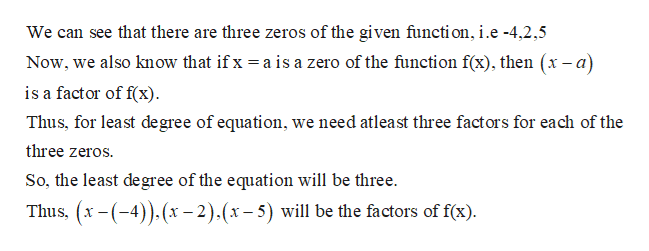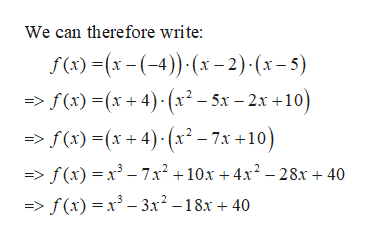# Write an equation of least degree with intergral coefficents that has the given zeros.-4, 2, 5Explanation please.

Question
1 views

Write an equation of least degree with intergral coefficents that has the given zeros.

-4, 2, 5

check_circle

Step 1

We know:help_outlineImage TranscriptioncloseWe can see that there are three zeros of the given functi on, i.e -4,2,5 Now, we also know that if x is a factor of f(x) a is a zero of the function f(x), then (x-a) Thus, for least degree of equation, we need atleast three factors for each of the three zeros So, the least degree of the equation will be three. Thus, x-(4))x-2).(x-5) will be the factors of f(x) fullscreen
Step 2

If we multiply all the factors, ...help_outlineImage TranscriptioncloseWe can therefore write: f ()x(4) x-2) (x-5) f(x)(x+4)(x2-5x-2x+10) f ()x+4)(x-7x +10) _ f(x)x3 - 7x2 +10x +4x2 -28x + 40 => f(x)x3-3x2-18x + 40 fullscreen

### Want to see the full answer?

See Solution

#### Want to see this answer and more?

Solutions are written by subject experts who are available 24/7. Questions are typically answered within 1 hour.*

See Solution
*Response times may vary by subject and question.
Tagged in

### Polynomials